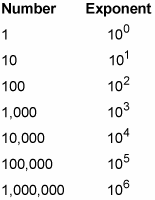##### Statistics: 1001 Practice Problems For Dummies (+ Free Online Practice)When working with extremely large or small numbers, you can save on time and space — and make calculations easier — by using scientific notation. As you will see, it uses both decimals and exponents to represent these numbers in a kind of shorthand.

Scientific notation uses powers of ten expressed as exponents, so you need a little background before you can jump in.

Numbers starting with a 1 and followed by only 0s (such 10, 100, 1,000, 10,000, and so forth) are called powers of ten, and they’re easy to represent as exponents. Powers of ten are the result of multiplying 10 times itself any number of times.

To represent a number that’s a power of 10 as an exponential number, count the zeros and raise 10 to that exponent. For example, 1,000 has three zeros, so 1,000 = 103 (103 means to take 10 times itself three times, so it equals 10 10 10). The following list shows some powers of ten expressed as exponents.After you know this trick, representing a lot of large numbers as powers of ten is easy — just count the 0s! For example, the number 1 trillion — 1,000,000,000,000 — is a 1 with twelve 0s after it, so

1,000,000,000,000 = 1012

This trick may not seem like a big deal, but the higher the numbers get, the more space you save by using exponents. For example, a really big number is a googol, which is 1 followed by a hundred 0s. You can write this as follows:

10,000,000,000,000,000,000,000,000,000,000,000,000,000,000,000,000,000, 000,000,000,000,000,000,000,000,000,000,000,000,000,000,000,000

As you can see, a number of this size is practically unmanageable. You can save yourself some trouble and write 10100.

A 10 raised to a negative number is also a power of ten. You can use negative exponents to represent decimals. For example,

10–1 = 0.1 10–2 = 0.01 10–3 = 0.001 10–4 = 0.0001

Although the idea of negative exponents may seem strange, it makes sense when you think about it alongside what you know about positive exponents. For example, to find the value of 107, start with 1 and make it larger by moving the decimal point 7 spaces to the right:

107 = 10,000,000

Similarly, to find the value of 10–7, start with 1 and make it smaller by moving the decimal point 7 spaces to the left:

10–7 = 0.0000001

Negative powers of 10 always have one fewer 0 between the 1 and the decimal point than the power indicates. In this example, notice that 10–7 has six 0s between the 1 and the decimal point.

As with very large numbers, using exponents to represent very small decimals makes practical sense. For example,

10–23 = 0.00000000000000000000001

As you can see, this decimal is easy to work with in its exponential form but almost impossible to read otherwise.